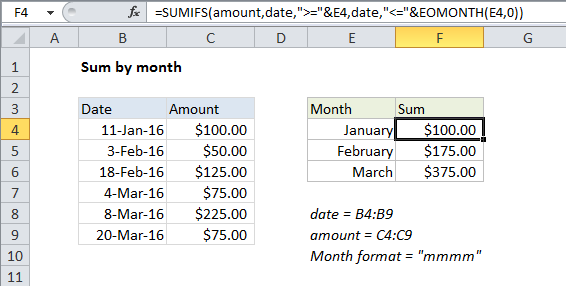## Excel Office

Excel How Tos, Tutorials, Tips & Tricks, Shortcuts

# Sum by month in Excel

This tutorial shows how to Sum by month in Excel using the example below;

## Formula

`=SUMIFS(values,date_range,">="&A1,date_range,"<="&EOMONTH(A1,0))`## Explanation

To sum by month, you can use a formula based on the SUMIFS function, with help from the EOMONTH function. In the example shown, the formula in F4 is:

`=SUMIFS(amount,date,">="&E4,date,"<="&EOMONTH(E4,0))`

This formula uses the named ranges “amount” (C4:C9) and “date” (B4:B9).

### How this formula works

The SUMIFS function can sum values in ranges based on multiple criteria.

In this case, we configure SUMIFS to sum amounts by month using two criteria: one to match dates greater than or equal to the first day of the month, one to match dates less than or equal to the last day of the month. In other words, we need a formula like this:

`=SUMIFS(amount,date,">="&"1/1/2016",date,"<="&"1/31/2016")`

Or the the more bulletproof alternative:

`=SUMIFS(amount,date,">="&DATE(2016,1,1),date,"<="&DATE(2016,1,31))`

This version is more robust because dates are constructed with separate year, month, and day values, and not entered as text that needs to be interpreted by Excel.

Worked Example:   Sum if not blank in Excel

Normally, this is a pain, because if you add month names as text (i.e. “January”, “February”, “March”, etc.) in column E you have to go to extra trouble to create dates you can use for criteria. However, in this case, we use a simple trick to make things easy: In column E, instead of typing month names, we add actual dates (1/1/2016, 2/1/2016, 3/1/2016), then use a custom date format (“mmmm”) to display the month names.

Worked Example:   Get last weekday in month in Excel

This makes it easy to build the criteria we need for SUMIFS. To match dates greater than or equal to the first of the month, we use:

`">="&E4`

And to match dates less than or equal to the last day of the month, we use this:

`"<="&EOMONTH(E4,0)`

With zero for months, the EOMONTH function automatically returns the last day of the month provided by the date.

Worked Example:   Sum if between in Excel

The concatenation with an ampersand (&) is necessary when building criteria that use a logical operator with a numeric value.